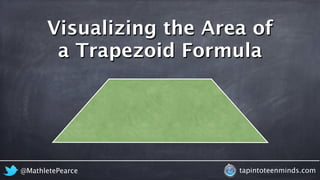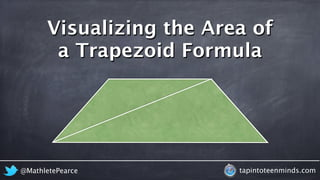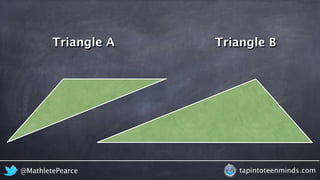Seu SlideShare está sendo baixado. ×

# Visualizing the Area of a Trapezoid Formula - Deriving the Algebraic Formula

Anúncio
Anúncio
Anúncio
Anúncio
Anúncio
Anúncio
Anúncio
Anúncio
Anúncio
Anúncio
AnúncioCarregando em…3
×

1 de 72 Anúncio

# Visualizing the Area of a Trapezoid Formula - Deriving the Algebraic Formula

Don't just just the formula; learn how the formula came to be! What better way to do that than by watching an animation that helps you visualize where the algebraic formula for finding the area of a trapezoid comes from?

Don't just just the formula; learn how the formula came to be! What better way to do that than by watching an animation that helps you visualize where the algebraic formula for finding the area of a trapezoid comes from?

Anúncio
Anúncio

### Visualizing the Area of a Trapezoid Formula - Deriving the Algebraic Formula

1. 1. Visualizing the Area of a Trapezoid Formula @MathletePearce tapintoteenminds.com
2. 2. Visualizing the Area of a Trapezoid Formula @MathletePearce tapintoteenminds.com
3. 3. Triangle A Triangle B @MathletePearce tapintoteenminds.com
4. 4. Area of Triangle A Area of Triangle B A = bh A = bh 2 2 @MathletePearce tapintoteenminds.com
5. 5. Area of Triangle A Area of Triangle B bh A = A = bh 2 2 base height height base @MathletePearce tapintoteenminds.com
6. 6. Area of Triangle A Area of Triangle B A = A = bh 2 bh 2 base base height height @MathletePearce tapintoteenminds.com
7. 7. Area of Triangle A Area of Triangle B A = A = bh 2 bh 2 base base height height @MathletePearce tapintoteenminds.com
8. 8. Area of Triangle A Area of Triangle B A = A = bh 2 bh 2 base base height height @MathletePearce tapintoteenminds.com
9. 9. Area of Triangle A Area of Triangle B A = A = bh 2 h a 2 base base height height a @MathletePearce tapintoteenminds.com
10. 10. Area of Triangle A Area of Triangle B A = A = b bh 2 h a 2 base base height height a b @MathletePearce tapintoteenminds.com
11. 11. Area of Triangle A Area of Triangle B A = A = b bh 2 h a 2 base base height height a b @MathletePearce tapintoteenminds.com
12. 12. Area of a Trapezoid Formula A = h ah b 2 2 + @MathletePearce tapintoteenminds.com
13. 13. Area of a Trapezoid Formula A = h b 2 h a 2 h a b + @MathletePearce tapintoteenminds.com
14. 14. Area of a Trapezoid Formula A = h b 2 h a 2 h a b + @MathletePearce tapintoteenminds.com
15. 15. Area of a Trapezoid Formula A = h b 2 h a 2 h a b + @MathletePearce tapintoteenminds.com
16. 16. Area of a Trapezoid Formula A = h b 2 h a 2 h a b + @MathletePearce tapintoteenminds.com
17. 17. Area of a Trapezoid Formula A = h b 2 h a ah 2 h a b + @MathletePearce tapintoteenminds.com
18. 18. Area of a Trapezoid Formula A = h b 2 h a 2 h a b + ah 2 @MathletePearce tapintoteenminds.com
19. 19. Area of a Trapezoid Formula A = h b 2 h a 2 h a b + ah 2 @MathletePearce tapintoteenminds.com
20. 20. Area of a Trapezoid Formula A = h b 2 h a 2 h a b + ah 2 @MathletePearce tapintoteenminds.com
21. 21. Area of a Trapezoid Formula A = h b bh 2 h a 2 h a b + ah 2 @MathletePearce tapintoteenminds.com
22. 22. Area of a Trapezoid Formula A = h b 2 h a 2 h a b + ah 2 bh 2 @MathletePearce tapintoteenminds.com
23. 23. Area of a Trapezoid Formula A = h b 2 h a 2 h a b + ah 2 bh 2 @MathletePearce tapintoteenminds.com
24. 24. Area of a Trapezoid Formula A = h ah b 2 2 + ah 2 bh 2 @MathletePearce tapintoteenminds.com
25. 25. Area of a Trapezoid Formula A = h ah b 2 2 + ah 2 bh 2 @MathletePearce tapintoteenminds.com
26. 26. Area of a Trapezoid Formula A = h ah b 2 2 + bh 2 ah 2 @MathletePearce tapintoteenminds.com
27. 27. Area of a Trapezoid Formula A = h ah b 2 2 + @MathletePearce tapintoteenminds.com
28. 28. Area of a Trapezoid Formula A = h ah b 2 2 + @MathletePearce tapintoteenminds.com
29. 29. Area of a Trapezoid Formula A = h ah b 2 2 + @MathletePearce tapintoteenminds.com
30. 30. Area of a Trapezoid Formula A = h ah b 2 2 + @MathletePearce tapintoteenminds.com
31. 31. Area of a Trapezoid Formula A = h ah b 2 2 + @MathletePearce tapintoteenminds.com
32. 32. Area of a Trapezoid Formula A = h ah b 2 2 + @MathletePearce tapintoteenminds.com
33. 33. Area of a Trapezoid Formula A = h ah b 2 2 + @MathletePearce tapintoteenminds.com
34. 34. Area of a Trapezoid Formula h a b h ah + b A = 2 2 @MathletePearce tapintoteenminds.com
35. 35. Area of a Trapezoid Formula h a b h ah + b A = 2 2 @MathletePearce tapintoteenminds.com
36. 36. Area of a Trapezoid Formula h a b A = h ah + b 2 @MathletePearce tapintoteenminds.com
37. 37. Area of a Trapezoid Formula h a b ah + bh A = 2 @MathletePearce tapintoteenminds.com
38. 38. A = Area of a Trapezoid Formula ah + bh 2 h a b @MathletePearce tapintoteenminds.com
39. 39. A = Area of a Trapezoid Formula ah + bh 2 @MathletePearce tapintoteenminds.com h a b
40. 40. A = Area of a Trapezoid Formula ah + bh 2 @MathletePearce tapintoteenminds.com h a b
41. 41. A = Area of a Trapezoid Formula ah + bh 2 @MathletePearce tapintoteenminds.com h a b
42. 42. A = Area of a Trapezoid Formula ah + bh 2 h a b @MathletePearce tapintoteenminds.com
43. 43. A = Area of a Trapezoid Formula ah + bh 2 @MathletePearce tapintoteenminds.com h a b
44. 44. A = Area of a Trapezoid Formula ah + bh 2 @MathletePearce tapintoteenminds.com h a b
45. 45. A = Area of a Trapezoid Formula ah + bh 2 @MathletePearce tapintoteenminds.com h a b
46. 46. A = Area of a Trapezoid Formula ah + bh 2 @MathletePearce tapintoteenminds.com
47. 47. A = Area of a Trapezoid Formula ah + bh 2 @MathletePearce tapintoteenminds.com
48. 48. A = Area of a Trapezoid Formula ah + bh 2 @MathletePearce tapintoteenminds.com
49. 49. A = Area of a Trapezoid Formula ah + bh 2 @MathletePearce tapintoteenminds.com
50. 50. A = Area of a Trapezoid Formula ah + bh 2 @MathletePearce tapintoteenminds.com
51. 51. A = Area of a Trapezoid Formula ah + bh 2 @MathletePearce tapintoteenminds.com
52. 52. A = Area of a Trapezoid Formula ah + bh 2 @MathletePearce tapintoteenminds.com
53. 53. A = Area of a Trapezoid Formula ah + bh 2 @MathletePearce tapintoteenminds.com
54. 54. A = Area of a Trapezoid Formula ah + bh 2 @MathletePearce tapintoteenminds.com
55. 55. A = Area of a Trapezoid Formula ah + bh 2 @MathletePearce tapintoteenminds.com
56. 56. A = Area of a Trapezoid Formula ah + bh 2 @MathletePearce tapintoteenminds.com
57. 57. A = Area of a Trapezoid Formula ah + bh 2 @MathletePearce tapintoteenminds.com
58. 58. A = Area of a Trapezoid Formula h ( a + b ) 2 @MathletePearce tapintoteenminds.com
59. 59. A = Area of a Trapezoid Formula h ( a + b ) 2 h a b @MathletePearce tapintoteenminds.com
60. 60. A = Area of a Trapezoid Formula h ( a + b ) 2 @MathletePearce tapintoteenminds.com h a b
61. 61. A = Area of a Trapezoid Formula h ( a + b ) 2 @MathletePearce tapintoteenminds.com h a b
62. 62. A = Area of a Trapezoid Formula h ( a + b ) 2 @MathletePearce tapintoteenminds.com
63. 63. A = Area of a Trapezoid Formula h ( a + b ) 2 @MathletePearce tapintoteenminds.com
64. 64. A = Area of a Trapezoid Formula h ( a + b ) 2 @MathletePearce tapintoteenminds.com
65. 65. A = Area of a Trapezoid Formula h ( a + b ) 2 @MathletePearce tapintoteenminds.com
66. 66. A = Area of a Trapezoid Formula h ( a + b ) 2 @MathletePearce tapintoteenminds.com
67. 67. A = Area of a Trapezoid Formula h ( a + b ) 2 @MathletePearce tapintoteenminds.com
68. 68. A = Area of a Trapezoid Formula h ( a + b ) 2 @MathletePearce tapintoteenminds.com
69. 69. A = Area of a Trapezoid Formula h ( a + b ) 2 @MathletePearce tapintoteenminds.com
70. 70. Area of a Trapezoid Formula A = h a b ( + ) 2 @MathletePearce tapintoteenminds.com
71. 71. Area of a Trapezoid Formula ah + bh ah + b h A = 2 A = 2 A = h a b ( + ) 2 2 @MathletePearce tapintoteenminds.com
72. 72. Area of a Trapezoid Formula ah + bh ah + b h A = 2 A = 2 A = h a b ( + ) 2 2 @MathletePearce tapintoteenminds.com